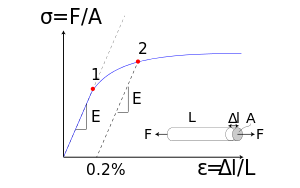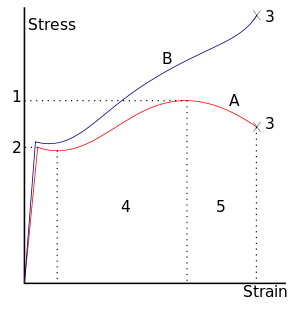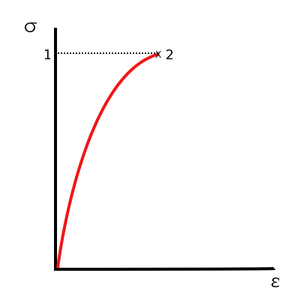# Stress–strain curveFig.1: Stress–strain curve showing typical yield behavior for nonferrous alloys. Stress () is shown as a function of strain ()
1: Elastic (proportionality) limit
2: Offset yield strength (0.2% proof strength)

The relationship between the stress and strain that a particular material displays is known as that particular material's stress–strain curve. It is unique for each material and is found by recording the amount of deformation (strain) at distinct intervals of tensile or compressive loading (stress). These curves reveal many of the properties of a material (including data to establish the Modulus of Elasticity, E).

Stress–strain curves of various materials vary widely, and different tensile tests conducted on the same material yield different results, depending upon the temperature of the specimen and the speed of the loading. It is possible, however, to distinguish some common characteristics among the stress–strain curves of various groups of materials and, on this basis, to divide materials into two broad categories; namely, the ductile materials and the brittle materials.

Consider a bar of cross sectional area A being subjected to equal and opposite forces F pulling at the ends so the bar is under tension. The material is experiencing a stress defined to be the ratio of the force to the cross sectional area of the bar:This stress is called the tensile stress because every part of the object is subjected to tension. The SI unit of stress is the newton per square meter, which is called the pascal.
1 pascal = 1 Pa = 1 N/m2

Now consider a force that is applied tangentially to an object. The ratio of the shearing force to the area A is called the shear stress.

If the object is twisted through an angle q, then the shear strain is:Finally, the shear modulus MS of a material is defined as the ratio of shear stress to shear strain at any point in an object made of that material. The shear modulus is also known as the torsion modulus.

## Ductile materialsA stress–strain curve typical of structural steel.

Ductile materials, which includes structural steel and many alloys of other metals, are characterized by their ability to yield at normal temperatures.

Low carbon steel generally exhibits a very linear stress–strain relationship up to a well defined yield point (Fig.2). The linear portion of the curve is the elastic region and the slope is the modulus of elasticity or Young's Modulus ( Young's Modulus is the ratio of the compressive stress to the longitudinal strain). After the yield point, the curve typically decreases slightly because of dislocations escaping from Cottrell atmospheres. As deformation continues, the stress increases on account of strain hardening until it reaches the ultimate tensile stress. Until this point, the cross-sectional area decreases uniformly and randomly because of Poisson contractions. The actual fracture point is in the same vertical line as the visual fracture point.

However, beyond this point a neck forms where the local cross-sectional area becomes significantly smaller than the original. The ratio of the tensile force to the true cross-sectional area at the narrowest region of the neck is called the true stress. The ratio of the tensile force to the original cross-sectional area is called the engineering stress. If the stress–strain curve is plotted in terms of true stress and true strain the stress will continue to rise until failure. Eventually the neck becomes unstable and the specimen fractures.

If the specimen is subjected to progressively increasing tensile force it reaches the ultimate tensile stress and then necking and elongation occur rapidly until fracture. If the specimen is subjected to progressively increasing length it is possible to observe the progressive necking and elongation, and to measure the decreasing tensile force in the specimen. Civil structures such as bridges are mostly loaded in a manner that applies progressively increasing forces. Sheet-metal pressing operations subject the work-piece to progressively increasing deformation. In order to accurately record the behavior of materials between ultimate tensile stress and fracture, most tensile-testing machines load the specimen with a progressively increasing length.

Less ductile materials such as medium to high carbon steels do not have a well-defined yield point. There are generally two types of yield point - upper and lower yield points. For these materials the yield stress is typically determined by the "offset yield method", by which a line is drawn parallel to the linear elastic portion of the curve and intersecting the abscissa at some arbitrary value (generally from 0.1% to 0.2%). The intersection of this line and the stress–strain curve is reported as the yield point. The elastic region is the portion of the curve where the material will return to its original shape if the load is removed. The plastic region is the portion where some permanent deformation will occur, even if the load is removed. Failure point is when the specimen fractures.Fig.3 Stress–strain curve for brittle materials.

## Brittle materials

Brittle materials, which includes cast iron, glass, and stone, are characterized by the fact that rupture occurs without any noticeable prior change in the rate of elongation.

Brittle materials such as concrete or carbon fiber do not have a yield point, and do not strain-harden. Therefore, the ultimate strength and breaking strength are the same. A typical stress–strain curve is shown in Fig.3. Typical brittle materials like glass do not show any plastic deformation but fail while the deformation is elastic. One of the characteristics of a brittle failure is that the two broken parts can be reassembled to produce the same shape as the original component as there will not be a neck formation like in the case of ductile materials. A typical stress–strain curve for a brittle material will be linear. For some materials, such as concrete, tensile strength is negligible compared to the compressive strength and it is assumed zero for many engineering applications. Glass fibers have a tensile strength stronger than steel, but bulk glass usually does not. This is because of the stress intensity factor associated with defects in the material. As the size of the sample gets larger, the size of defects also grows. In general, the tensile strength of a rope is always less than the sum of the tensile strengths of its individual fibers.